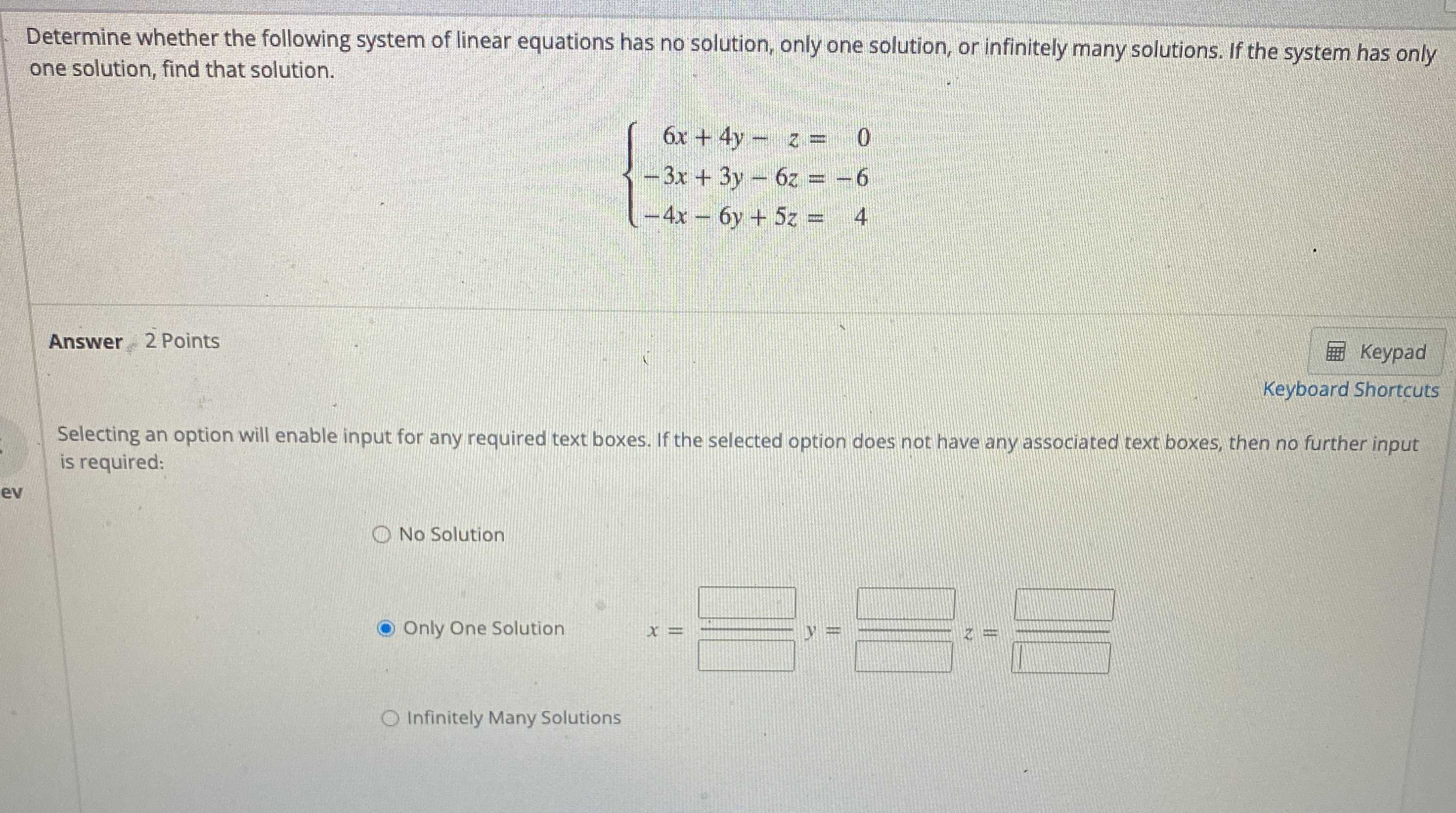### Still have math questions?

Algebra
QuestionDetermine whether the following system of linear equations has no solution, only one solution, or infinitely many solutions. If the system has only one solution, find that solution.

$$\left\{ \begin{array} { r } { 6 x + 4 y - z = 0 } \\ { - 3 x + 3 y - 6 z = - 6 } \\ { - 4 x - 6 y + 5 z = 4 } \end{array} \right.$$